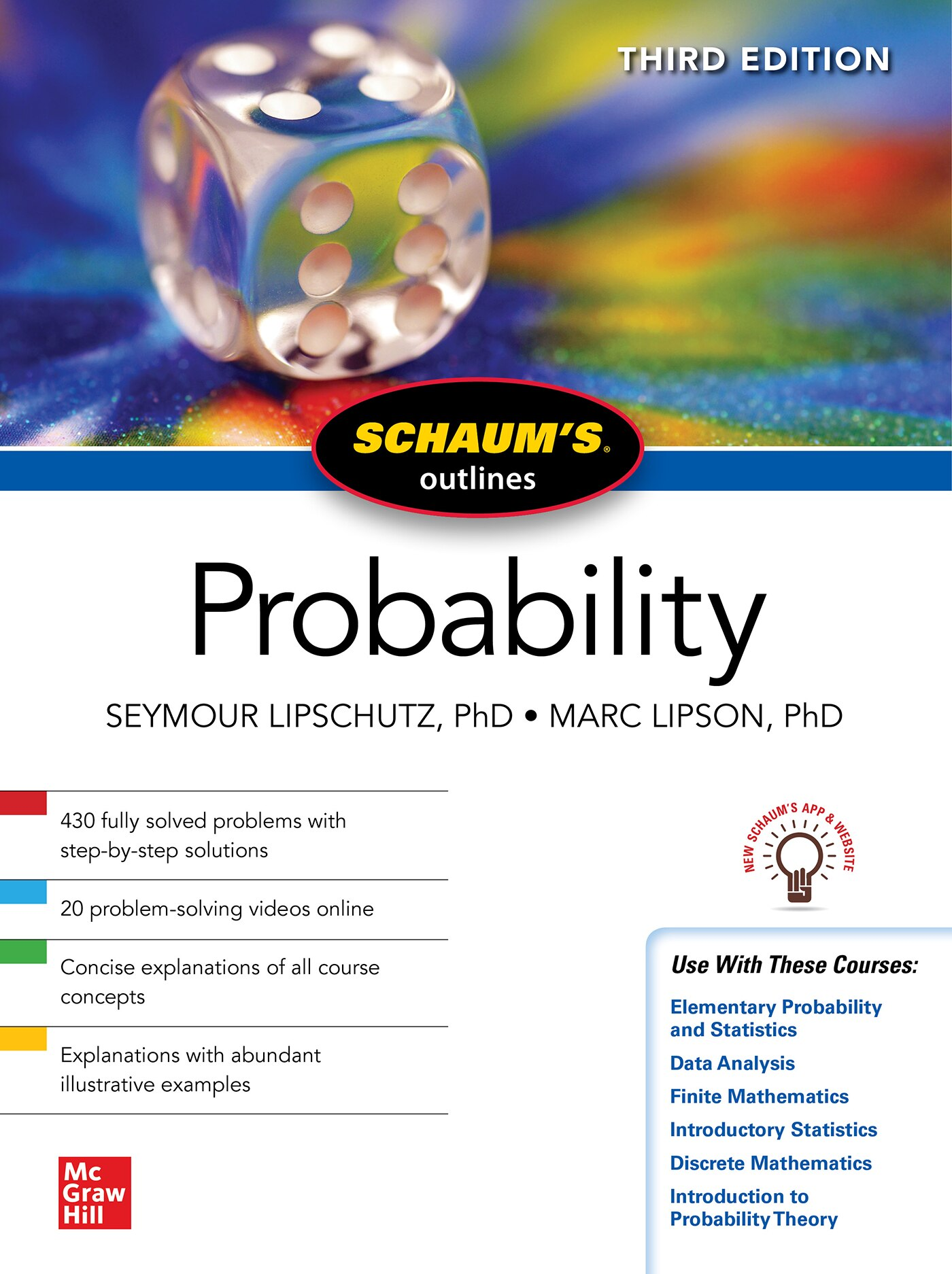# Schaum's Outline of Probability, Third Edition

## bySeymour Lipschutz, Marc Lipson

November 12, 2021|\$29.95
Paperback
Earn 150 plum® points
Free shipping on orders over \$35
Pick up in store
To see if pickup is available,
Find In Store
Not sold in stores
Prices and offers may vary in store

Study smarter and stay on top of your probability course with the bestselling Schaum''s Outline-now with the NEW Schaum''s app and website!

Schaum''s Outline of Probability, Third Edition is the go-to study guide for help in probability courses. It''s ideal for undergrads, graduate students and professionals needing a tool for review. With an outline format that facilitates quick and easy review and mirrors the course in scope and sequence, this book helps you understand basic concepts and get the extra practice you need to excel in the course. Schaum''s Outline of Probability, Third Edition supports the bestselling textbooks and is useful for a variety of classes, including Elementary Probability and Statistics, Data Analysis, Finite Mathematics, and many other courses.

You''ll find coverage on finite and countable sets, binomial coefficients, axioms of probability, conditional probability, expectation of a finite random variable, Poisson distribution, and probability of vectors and Stochastic matrices. Also included: finite Stochastic and tree diagrams, Chebyshev''s inequality and the law of large numbers, calculations of binomial probabilities using normal approximation, and regular Markov processes and stationary state distributions.

Features

.NEW to this edition: the new Schaum''s app and website!
.NEW to this edition: 20 NEW problem-solving videos online
.430 solved problems
.Outline format to provide a concise guide to the standard college course in probability
.Clear, concise explanations of probability concepts
.Supports these major texts: Elementary Statistics: A Step by Step Approach (Bluman), Mathematics with Applications (Hungerford), and Discrete Mathematics and Its Applications (Rosen)
.Appropriate for the following courses: Elementary Probability and Statistics, Data Analysis, Finite Mathematics, Introduction to Mathematical Statistics, Mathematics for Biological Sciences, Introductory Statistics, Discrete Mathematics, Probability for Applied Science, and Introduction to Probability Theory

Seymour Lipschutz, Ph.D., was on the mathematics faculty of Temple University and previously taught at the Polytechnic Institute of Brooklyn. He was also a visiting professor in the Computer Science Department of Brooklyn College. His work in the Schaum''s Outline series includes Beginning Linear Algebra, Discrete Mathem...